# Practice Test: Pie Chart - 1

## 5 Questions MCQ Test Quantitative Aptitude for Banking Preparation | Practice Test: Pie Chart - 1

Description
Attempt Practice Test: Pie Chart - 1 | 5 questions in 10 minutes | Mock test for UPSC preparation | Free important questions MCQ to study Quantitative Aptitude for Banking Preparation for UPSC Exam | Download free PDF with solutions
QUESTION: 1

### The following pie-chart shows the percentage distribution of the expenditure incurred in publishing a book. Study the pie-chart and the answer the questions based on it.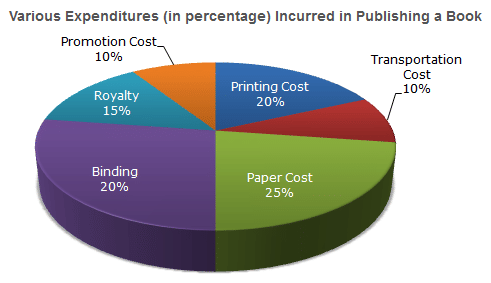If for a certain quantity of books, the publisher has to pay Rs. 30,600 as printing cost, then what will be amount of royalty to be paid for these books?

Solution:

Let the amount of Royalty to be paid for these books be Rs. r.
Then 20:15 = 30600: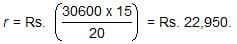QUESTION: 2

### The following pie-chart shows the percentage distribution of the expenditure incurred in publishing a book. Study the pie-chart and the answer the questions based on it.What is the central angle of the sector corresponding to the expenditure incurred on Royalty?

Solution:

Central angle corresponding to Royalty  = (15% of 360)0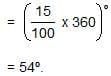QUESTION: 3

### The following pie-chart shows the percentage distribution of the expenditure incurred in publishing a book. Study the pie-chart and the answer the questions based on it.The price of the book is marked 20% above the C.P. If the marked price of the book is Rs. 180, then what is the cost of the paper used in a single copy of the book?

Solution:

Clearly, marked price of the book = 120% of C.P
Also  cost of paper = 25% of C.P
Let the  cost of paper for a single book be Rs. n.
Then, 120 : 25 = 180 : n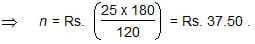QUESTION: 4

The following pie-chart shows the percentage distribution of the expenditure incurred in publishing a book. Study the pie-chart and the answer the questions based on it.If 5500 copies are published and the transportation cost on them amounts to Rs. 82500, then what should be the selling price of the book so that the publisher can earn a profit of 25%?

Solution:

For  the  publisher to earn a profit of  25% S.P. = 125% of C.P.
Also  Transportation Cost = 10% of C.P.
Let the S.P. of 5500 books beRs.x.
Then, 10 : 125 = 82500 : x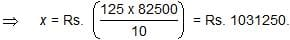∴ S.P. of  one  book = Rs.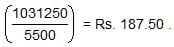QUESTION: 5

The following pie-chart shows the percentage distribution of the expenditure incurred in publishing a book. Study the pie-chart and the answer the questions based on it.Royalty on the book is less than the printing cost by:

Solution: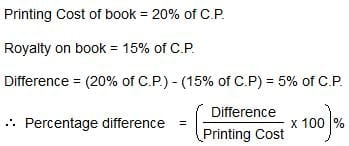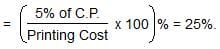Use Code STAYHOME200 and get INR 200 additional OFF Use Coupon Code